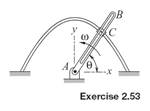### Create an Account

Home / Questions / Collar C is pushed along the guide bar defined by y 21 0252 where x and y have units of m...

# Collar C is pushed along the guide bar defined by y 21 0252 where x and y have units of meters The angular speed of arm AB that actuates the motion is the constant value

Collar C is pushed along the guide bar defined by y = 2(1 − 0.25x2), where x and y have units of meters. The angular speed of arm AB that actuates the motion is the constant value ω = 20 rad/s, so θ = ωt. Determine the forces exerted on the collar by arm AB and the guide bar at x = 1 m. The mass of the collar is 2 kg, and gravitational effects are ignorable.Jun 18 2020 View more View LessSubscribe To Get Solution